# Ballspring model of a bond Lattice Cubic lattice

• Slides: 23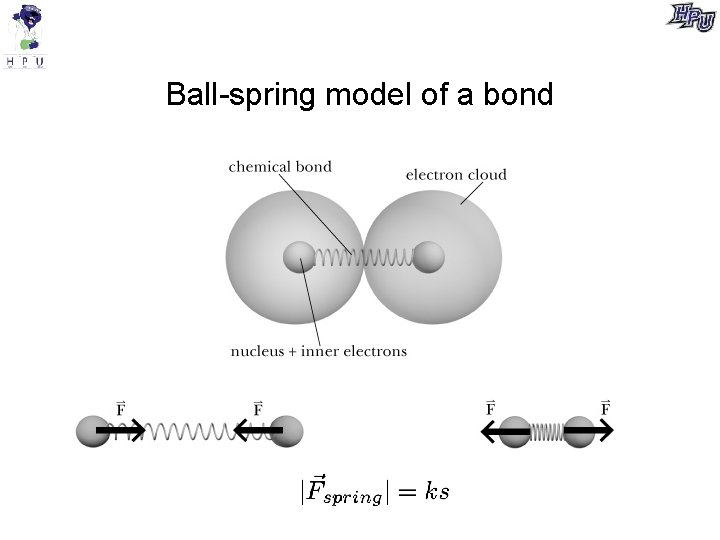Ball-spring model of a bondLattice Cubic latticeTension Use a 1 -D ball-spring model of a solid to describe forces within a wire, string, chain, rope, etc. This atomic force of the “spring” on an atom is called tension.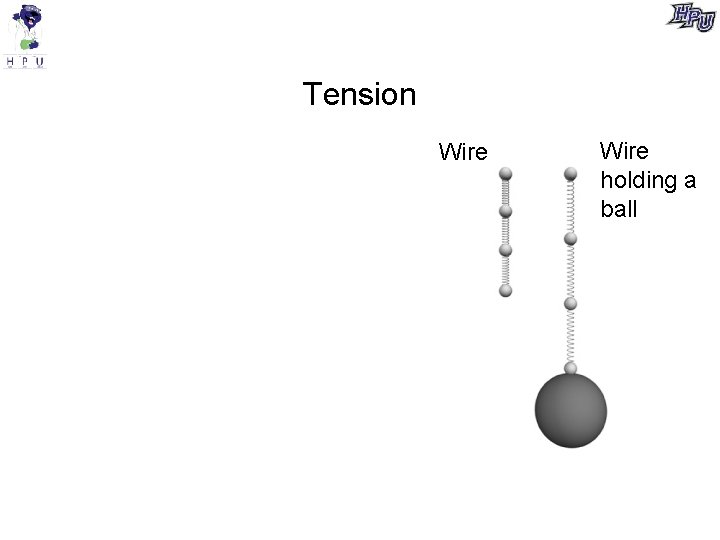Tension Wire holding a ball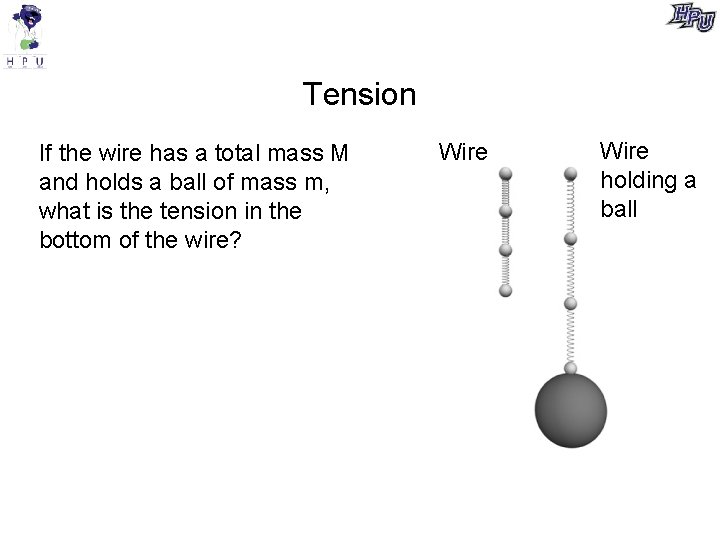Tension If the wire has a total mass M and holds a ball of mass m, what is the tension in the bottom of the wire? Wire holding a ballTension If the wire has a total mass M and holds a ball of mass m, what is the tension in the top of the wire? Wire holding a ballPoll A 10 -kg object hangs from a 1 -kg wire. The tension at the bottom of the wire is the tension at the top of the wire. 1. Greater than 2. Less than 3. Equal to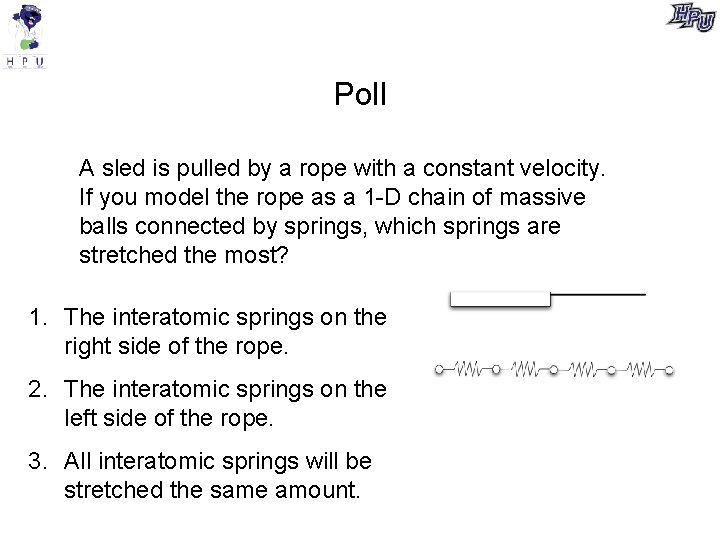Poll A sled is pulled by a rope with a constant velocity. If you model the rope as a 1 -D chain of massive balls connected by springs, which springs are stretched the most? 1. The interatomic springs on the right side of the rope. 2. The interatomic springs on the left side of the rope. 3. All interatomic springs will be stretched the same amount.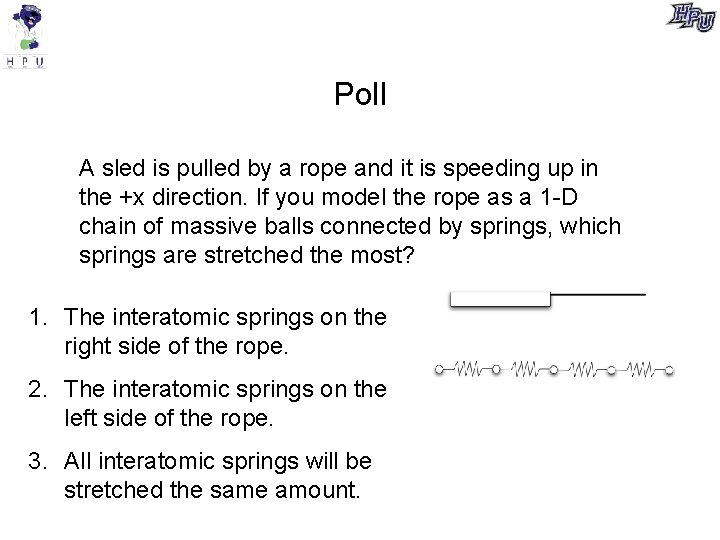Poll A sled is pulled by a rope and it is speeding up in the +x direction. If you model the rope as a 1 -D chain of massive balls connected by springs, which springs are stretched the most? 1. The interatomic springs on the right side of the rope. 2. The interatomic springs on the left side of the rope. 3. All interatomic springs will be stretched the same amount.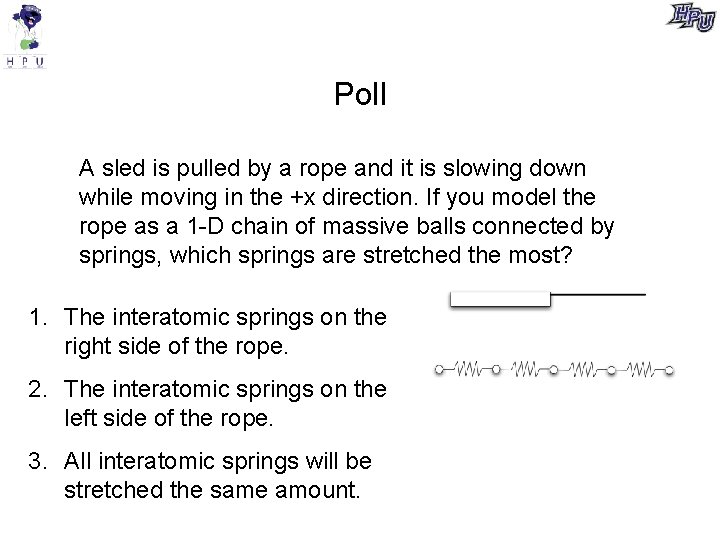Poll A sled is pulled by a rope and it is slowing down while moving in the +x direction. If you model the rope as a 1 -D chain of massive balls connected by springs, which springs are stretched the most? 1. The interatomic springs on the right side of the rope. 2. The interatomic springs on the left side of the rope. 3. All interatomic springs will be stretched the same amount.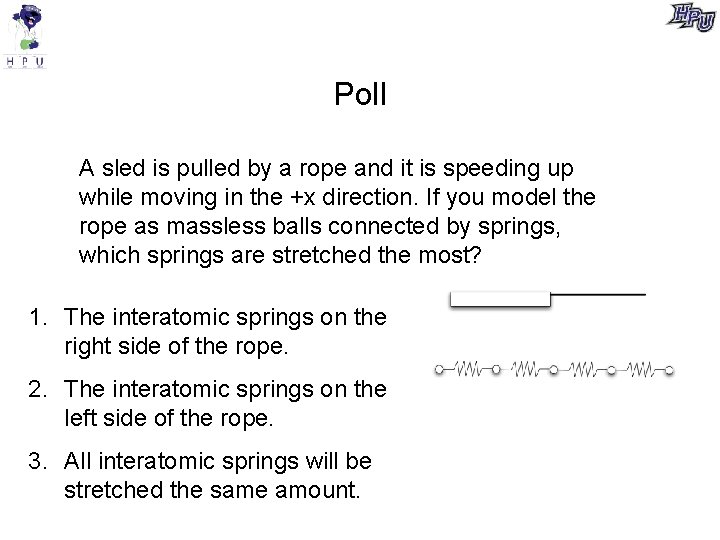Poll A sled is pulled by a rope and it is speeding up while moving in the +x direction. If you model the rope as massless balls connected by springs, which springs are stretched the most? 1. The interatomic springs on the right side of the rope. 2. The interatomic springs on the left side of the rope. 3. All interatomic springs will be stretched the same amount.A better model of a wire is a 2 -D array of 1 -D spring-ball chains.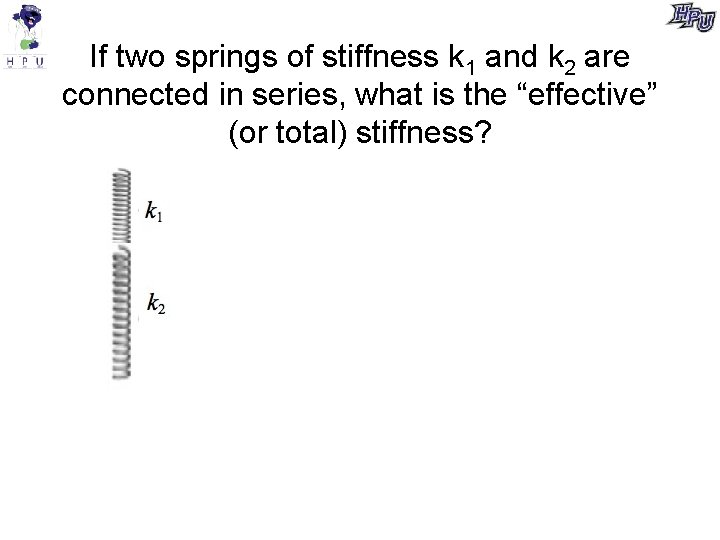If two springs of stiffness k 1 and k 2 are connected in series, what is the “effective” (or total) stiffness?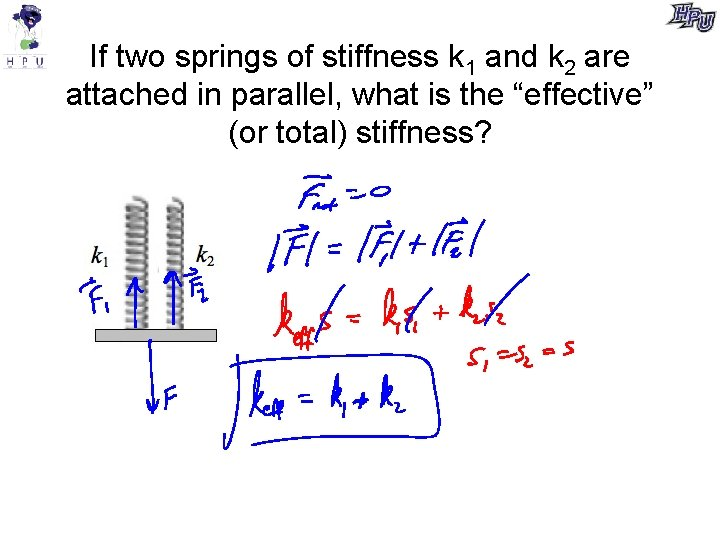If two springs of stiffness k 1 and k 2 are attached in parallel, what is the “effective” (or total) stiffness?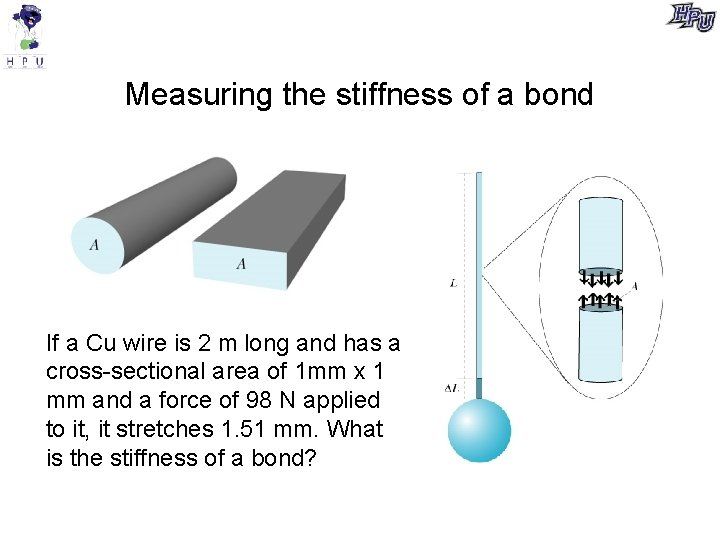Measuring the stiffness of a bond If a Cu wire is 2 m long and has a cross-sectional area of 1 mm x 1 mm and a force of 98 N applied to it, it stretches 1. 51 mm. What is the stiffness of a bond?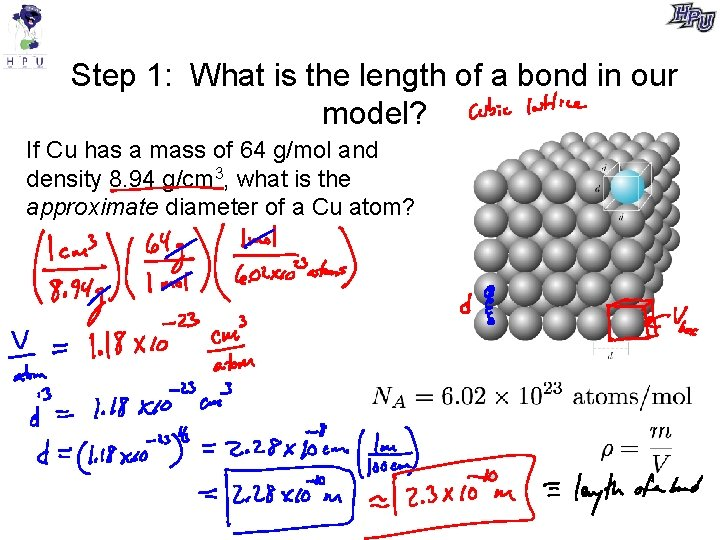Step 1: What is the length of a bond in our model? If Cu has a mass of 64 g/mol and density 8. 94 g/cm 3, what is the approximate diameter of a Cu atom?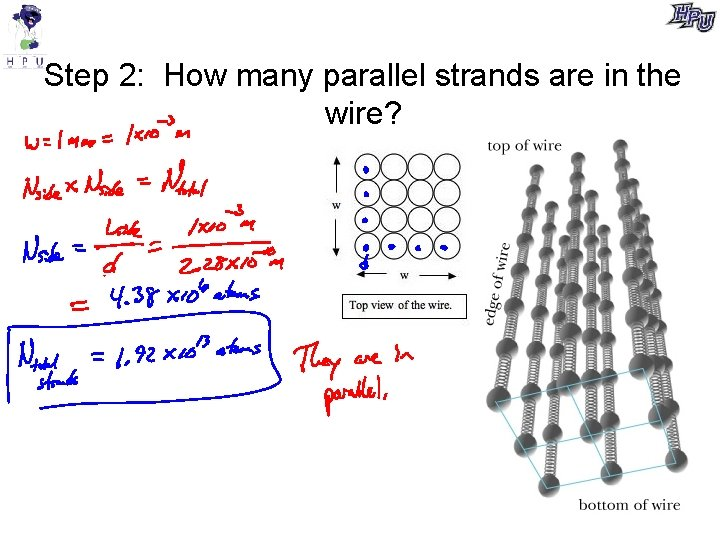Step 2: How many parallel strands are in the wire?Step 4: How many atoms are along the length of the wire?Step 5: What is the stiffness of a single spring in our model?Young’s modulus A macroscopically measured constant that is related to the stiffness of the bonds in a solid (microscopic). Units are N/m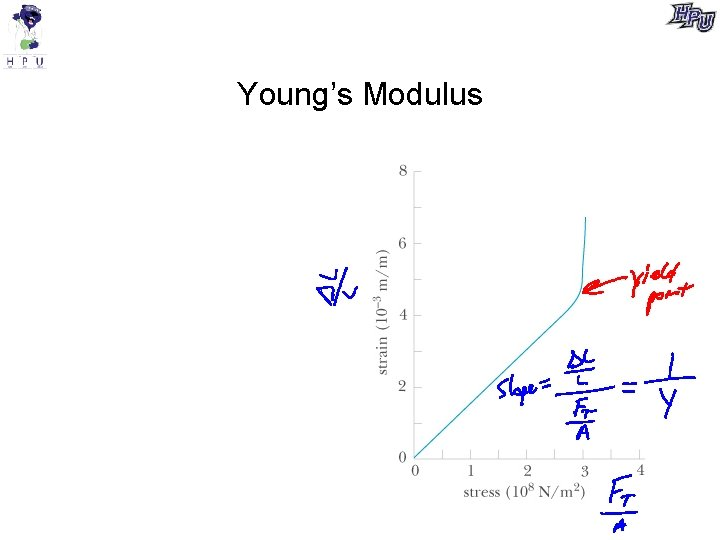Young’s Modulus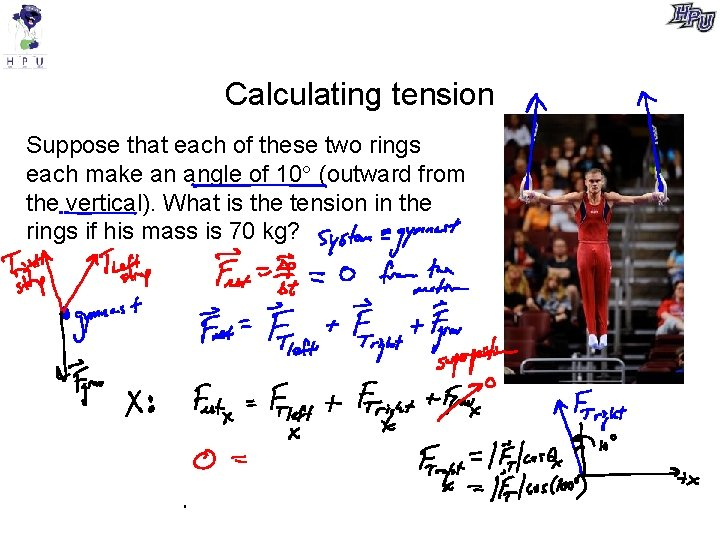Calculating tension Suppose that each of these two rings each make an angle of 10 (outward from the vertical). What is the tension in the rings if his mass is 70 kg?